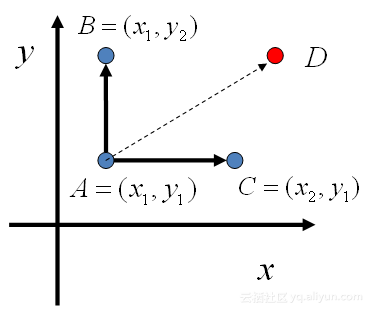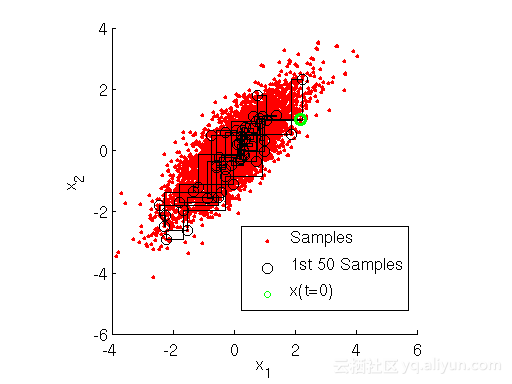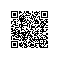# MCMC(四)Gibbs采样

1. 重新寻找合适的细致平稳条件

$$\pi(i)P(i,j) = \pi(j)P(j,i)$$

$$\pi(x_1^{(1)},x_2^{(1)}) \pi(x_2^{(2)} | x_1^{(1)}) = \pi(x_1^{(1)})\pi(x_2^{(1)}|x_1^{(1)}) \pi(x_2^{(2)} | x_1^{(1)})$$

$$\pi(x_1^{(1)},x_2^{(2)}) \pi(x_2^{(1)} | x_1^{(1)}) = \pi(x_1^{(1)}) \pi(x_2^{(2)} | x_1^{(1)})\pi(x_2^{(1)}|x_1^{(1)})$$

$$\pi(x_1^{(1)},x_2^{(1)}) \pi(x_2^{(2)} | x_1^{(1)}) = \pi(x_1^{(1)},x_2^{(2)}) \pi(x_2^{(1)} | x_1^{(1)})$$

$$\pi(A) \pi(x_2^{(2)} | x_1^{(1)}) = \pi(B) \pi(x_2^{(1)} | x_1^{(1)})$$

$$\pi(A) \pi(x_1^{(2)} | x_2^{(1)}) = \pi(C) \pi(x_1^{(1)} | x_2^{(1)})$$$$P(A \to B) = \pi(x_2^{(B)}|x_1^{(1)})\;\; if\; x_1^{(A)} = x_1^{(B)} =x_1^{(1)}$$

$$P(A \to C) = \pi(x_1^{(C)}|x_2^{(1)})\;\; if\; x_2^{(A)} = x_2^{(C)} =x_2^{(1)}$$

$$P(A \to D) = 0\;\; else$$

$$\pi(E)P(E \to F) = \pi(F)P(F \to E)$$

2. 二维Gibbs采样

1. 输入平稳分布$\pi(x_1,x_2)$，设定状态转移次数阈值$n_1$，需要的样本个数$n_2$
2. 随机初始化初始状态值$x_1^{(0)}$和$x_2^{(0)}$
3. for $t = 0$ to $n_1 +n_2-1$:

1. 从条件概率分布$P(x_2|x_1^{(t)})$中采样得到样本$x_2^{t+1}$
2. 从条件概率分布$P(x_1|x_2^{(t+1)})$中采样得到样本$x_1^{t+1}$

$$(x_1^{(1)}, x_2^{(1)}) \to (x_1^{(1)}, x_2^{(2)}) \to (x_1^{(2)}, x_2^{(2)}) \to ... \to (x_1^{(n_1+n_2-1)}, x_2^{(n_1+n_2-1)})$$3. 多维Gibbs采样

1. 输入平稳分布$pi(x_1,x_2，...,x_n)$或者对应的所有特征的条件概率分布，设定状态转移次数阈值$n_1$，需要的样本个数$n_2$
2. 随机初始化初始状态值$(x_1^{(0)},x_2^{(0)},...,x_n^{(0)})$
3. for $t = 0$ to $n_1 +n_2-1$:

1. 从条件概率分布$P(x_1|x_2^{(t)}, x_3^{(t)},...,x_n^{(t)})$中采样得到样本$x_1^{t+1}$
2. 从条件概率分布$P(x_2|x_1^{(t+1)}, x_3^{(t)}, x_4^{(t)},...,x_n^{(t)})$中采样得到样本$x_2^{t+1}$
3. ...
4. 从条件概率分布$P(x_j|x_1^{(t+1)}, x_2^{(t+1)},..., x_{j-1}^{(t+1)},x_{j+1}^{(t)}...,x_n^{(t)})$中采样得到样本$x_j^{t+1}$
5. ...
6. 从条件概率分布$P(x_n|x_1^{(t+1)}, x_2^{(t+1)},...,x_{n-1}^{(t+1)})$中采样得到样本$x_n^{t+1}$使用钉钉扫一扫加入圈子
+ 订阅